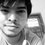# Why is a negative number multiplied by a negative number, a positive number?

Let's say you are playing a game involving black and red chips. At the end of the game, for each black chip that you have, you receive one dollar $+1$/extract_itex]. For each red chip that you have, you have to pay one dollar $-1\$. Now, these chips are packed together in bags of five, and say at some point in the game you've got several bags of black chips and several bags of red chips. If someone gives you three bags of black chips, then you gain 15 dollars. $3 \times 5 =15$. If someone takes away three of your bags of black chips, then you lose 15 dollars. $-3 \times 5=-15$. If someone gives you three bags of red chips, then you lose 15 dollars. $3 \times-5=-15$. If someone takes away three of your bags of red chips, then you gain 15 dollars. $-3 \times -5=15$. The key idea is that negative numbers represent changes, not amounts. It doesn't make sense to say that you have $-4$ slices of bread. It does, however, make sense to say that you ate $4$ slices of bread, and therefore the change in the number of slices you have is $-4$. This article is a copy from the notes of a PhD student of MIT. The original post is here !Note by Sayan Chaudhuri 5 years, 3 months ago This discussion board is a place to discuss our Daily Challenges and the math and science related to those challenges. Explanations are more than just a solution — they should explain the steps and thinking strategies that you used to obtain the solution. Comments should further the discussion of math and science. When posting on Brilliant: • Use the emojis to react to an explanation, whether you're congratulating a job well done , or just really confused . • Ask specific questions about the challenge or the steps in somebody's explanation. Well-posed questions can add a lot to the discussion, but posting "I don't understand!" doesn't help anyone. • Try to contribute something new to the discussion, whether it is an extension, generalization or other idea related to the challenge. • Stay on topic — we're all here to learn more about math and science, not to hear about your favorite get-rich-quick scheme or current world events. MarkdownAppears as *italics* or _italics_ italics **bold** or __bold__ bold - bulleted- list • bulleted • list 1. numbered2. list 1. numbered 2. list Note: you must add a full line of space before and after lists for them to show up correctly paragraph 1paragraph 2 paragraph 1 paragraph 2 [example link](https://brilliant.org)example link > This is a quote This is a quote  # I indented these lines # 4 spaces, and now they show # up as a code block. print "hello world" # I indented these lines # 4 spaces, and now they show # up as a code block. print "hello world" MathAppears as Remember to wrap math in $$ ... $$ or \[ ... $ to ensure proper formatting.
2 \times 3 $2 \times 3$
2^{34} $2^{34}$
a_{i-1} $a_{i-1}$
\frac{2}{3} $\frac{2}{3}$
\sqrt{2} $\sqrt{2}$
\sum_{i=1}^3 $\sum_{i=1}^3$
\sin \theta $\sin \theta$
\boxed{123} $\boxed{123}$

Sort by:

I don't know about the historic development of negative numbers. I would like to know what prompted Mathematicians to introduce them. But the concept of "debts" seems likely to be a cause of motivation or did benefit a lot as processing such matters, through negative numbers, became more effective. Amazing thing to note is that Mathematics, in this case, provided an effective notation rather than a new concept.

:)

- 5 years, 3 months ago

Lets make an assumption that you have a debt of 54shillings that means its negatively incurs you expenses on a monetary scope. If incur another debt. One will need to add more money to pay debt So debt of debt is increase in money. needed

- 5 years, 3 months ago